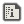Nintendo shutting down 3DS + Wii U online services, see our post

# String MappingRNGesusCreated:
THIS IS OBSOLETE AND I RECOMMEND THAT YOU SHOULD NEVER DO THIS! THERE ARE BETTER WAYS! Last night (March 3rd, 2016), I found out how to store map data into a TXT file. There are so many reasons to use TXT instead of DAT for storing map data, but with every way of doing something, there are always cons to using it. Here are the pros and cons of using TXT files to store map data. Pros:
• *You can decide to load a part of the map
• *It's easy to understand
• *It's perfect to use if you want custom maps in your game (like Super Mario Maker)
• *It has virtually infinite amount of separate characters to save the map with
• *You can save more than one layer of a map in the TXT
• *You are able to save other things in the TXT as well as the map data (such as the map's name)
• *It's easy to extract data
• *There are many more pros to using this, but I can't name them all
Cons:
• *Takes up more space
Now, onto the good stuff. Saving a map as a TXT file:
```FOR BGY=0 TO 63
FOR BGX=0 TO 63
NUM=BGGET(0,BGX,BGY,0)
MAPDATA\$=MAPDATA\$+CHR\$(NUM+14)
NEXT
NEXT
FOR BGY=0 TO 63
FOR BGX=0 TO 63
NUM=BGGET(1,BGX,BGY,0)
MAPDATA\$=MAPDATA\$+CHR\$(NUM+14)
NEXT
NEXT
SAVE"TXT:TESTMAP",MAPDATA\$
```
This code was made specifically for a 64x64 map, but you can change it to what ever size you like. Anyways, what this does is scans the screen for the BG tiles and converts it into a string. It scans each tile one at a time, but it's actually really fast. The CHR\$(13) bug is also avoided completely due to it increasing the CHR\$() by 14. Simple right?! I almost forgot to mention, you have to have BG tiles on the screen in order to save it as a TXT file. Therefore, you can make a map and save it using DAT and then load it and save it as a TXT. Loading a map from a TXT file:
```LOAD"TXT:TESTMAP",0 OUT MAPDATA\$
BGSCREEN 0,127,127
BGSCREEN 1,127,127

FOR NUM=0 TO 4096
BGPUT 0,BGX,BGY,ASC(MAPDATA\$[NUM])-14
BGX=BGX+1
IF BGX>=64 THEN BGY=BGY+1:BGX=0
BGNUM=0
NEXT
BGX=0:BGY=0:NUM=4095
FOR NUM=4095 TO 8192
BGPUT 0,BGX,BGY,ASC(MAPDATA\$[NUM])-14
BGX=BGX+1
IF BGX>=64 THEN BGY=BGY+1:BGX=0
BGNUM=0
NEXT
```
Ok, now things get a little bit more complicated but it's still simple. What this does is it loads the TXT file we previously made and then takes one character out of the string at a time, scans them, and then puts them in their proper place. As you can see, string mapping is quite simple. I initially made this so i can extract map collision data, but quickly found other uses for it. I hope you found this useful!!! I have begun remaking this engine for my new game on PC. I'll post a tech demo of both the PC and SB versions of this engine sometime....I did
```FOR I = 0 TO 65535
IF ASC(CHR\$(I)) ==I THEN ?"UNMAPPABLE",I
NEXT
```
nothing prints, indicating that asc is returning the correct value(0-65535). Anything over 65536 and asc doesn't reflect the "i" properly. so the whole for loop... FOR BGNUM=0 TO 16384 IF MAP\$==CHR\$(BGNUM) THEN BGPUT 1,BGX,BGY,(BGNUM-14):BGNUM=16384 NEXT probably can just be changed to BGPUT 1,BGX,BGY,asc(map\$)-14 and bgnum can be tossed. Ya probably already did that, and that's where you picked up the speed boost, and this post is old copy pastaReplying to:incvoid
...I did
```FOR I = 0 TO 65535
IF ASC(CHR\$(I)) ==I THEN ?"UNMAPPABLE",I
NEXT
```
nothing prints, indicating that asc is returning the correct value(0-65535). Anything over 65536 and asc doesn't reflect the "i" properly. so the whole for loop... FOR BGNUM=0 TO 16384 IF MAP\$==CHR\$(BGNUM) THEN BGPUT 1,BGX,BGY,(BGNUM-14):BGNUM=16384 NEXT probably can just be changed to BGPUT 1,BGX,BGY,asc(map\$)-14 and bgnum can be tossed. Ya probably already did that, and that's where you picked up the speed boost, and this post is old copy pasta
I tried that, but a 64*64 two layered map has too much information for that (over 8000 characters).I was able to increase the loading speed tenfold, thanks to incvoid and 12me21 for helping me out. EDIT:Because of this, I can make the maps much, much, much larger in the game I'm making.Optimization idea: You have
```FOR BGY=0 TO 63
FOR BGX=0 TO 63
NUM=BGGET(0,BGX,BGY,0)
MAPDATA\$=MAPDATA\$+CHR\$(NUM+14)
NEXT
NEXT
FOR BGY=0 TO 63
FOR BGX=0 TO 63
NUM=BGGET(1,BGX,BGY,0)
MAPDATA\$=MAPDATA\$+CHR\$(NUM+14)
NEXT
NEXT
SAVE"TXT:TESTMAP",MAPDATA\$```
but if you combine the two loops with another FOR statement:
```FOR LYR=0 TO 1
FOR BGY=0 TO 63
FOR BGX=0 TO 63
NUM=BGGET(LYR,BGX,BGY,0)
MAPDATA\$=MAPDATA\$+CHR\$(NUM+14)
NEXT
NEXT
NEXT
SAVE"TXT:TESTMAP",MAPDATA\$```
it can accomplish the same thing with less code. Just a thought :)Replying to:Minxrod
Optimization idea: You have
```FOR BGY=0 TO 63
FOR BGX=0 TO 63
NUM=BGGET(0,BGX,BGY,0)
MAPDATA\$=MAPDATA\$+CHR\$(NUM+14)
NEXT
NEXT
FOR BGY=0 TO 63
FOR BGX=0 TO 63
NUM=BGGET(1,BGX,BGY,0)
MAPDATA\$=MAPDATA\$+CHR\$(NUM+14)
NEXT
NEXT
SAVE"TXT:TESTMAP",MAPDATA\$```
but if you combine the two loops with another FOR statement:
```FOR LYR=0 TO 1
FOR BGY=0 TO 63
FOR BGX=0 TO 63
NUM=BGGET(LYR,BGX,BGY,0)
MAPDATA\$=MAPDATA\$+CHR\$(NUM+14)
NEXT
NEXT
NEXT
SAVE"TXT:TESTMAP",MAPDATA\$```
it can accomplish the same thing with less code. Just a thought :)
I already know about that. I used that way to make it a user defined command. Either way, you'll have to run it multiple times.ill try it this is perfect, im trying a metroid maker! thx ill try it!```DIM MAPDATA
BGSAVE 0,MAPDATA
SAVE "DAT:MAP",MAPDATA```
```DIM MAPDATAReplying to:12Me21
```DIM MAPDATA
```DIM MAPDATA